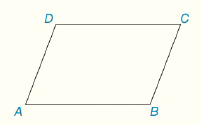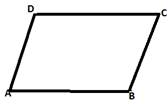# Consider ▱ ABCD as shown. a) How are ∠ A and ∠ C related? b) How are ∠ A and ∠ B related?### Elementary Geometry For College St...

7th Edition
Alexander + 2 others
Publisher: Cengage,
ISBN: 9781337614085### Elementary Geometry For College St...

7th Edition
Alexander + 2 others
Publisher: Cengage,
ISBN: 9781337614085

#### Solutions

Chapter
Section
Chapter 4.CT, Problem 1CT
Textbook Problem

## Consider ▱ ABCD as shown.a) How are ∠ A and ∠ C related?b) How are ∠ A and ∠ B related?Expert Solution
To determine

To Find:

The relation between A and C.

Solution:

A and C are congruent angles.

### Explanation of Solution

Given parallelogram,From the property opposite angles in a parallelogram are congruent.

Since ABCD is a parallelogram, A and C are opposite angles then A and C are congruent angles.

The Final statement:

A and C are congruent angles.

Expert Solution
To determine

To Find:

The relation between A and B.

Solution:

A and B are supplementary angles.

### Explanation of Solution

Given parallelogram,From the property consecutive angles in a parallelogram are supplementary.

Since ABCD is a parallelogram, A and B are consecutive angles then A and B are supplementary angles.

The Final statement:

A and B are supplementary angles.

### Want to see more full solutions like this?

Subscribe now to access step-by-step solutions to millions of textbook problems written by subject matter experts!

Get Solutions

### Want to see more full solutions like this?

Subscribe now to access step-by-step solutions to millions of textbook problems written by subject matter experts!

Get Solutions